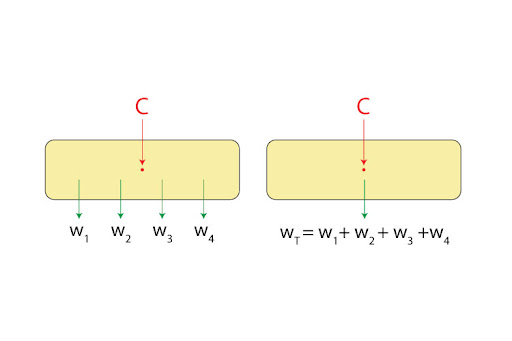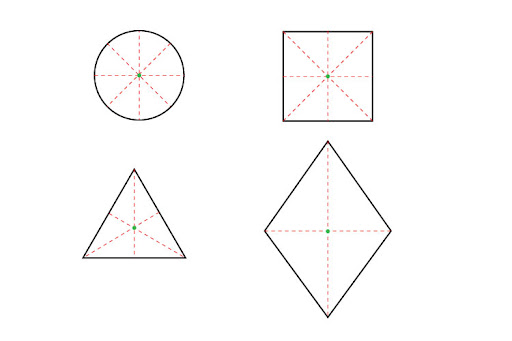# What is the 'centre of gravity' of an object and how do I calculate it?

The ‘centre of gravity’ is a point through which the entire weight of an object seems to act through. I say ‘seem to’ because in reality, the object’s weight would be distributed throughout its mass at different amounts. However, there will always exist one point which, once pivoted, would be a point of equilibrium - i.e. at that point, all the components of weight would create a zero net moment. It is equivalent to the scenario in which the entire weight of the object were to act straight through this pivoted center, such that no turning effect is produced.For a uniform - or regular - object, this is simply going to be the geometric center of its shape. Interesting thing to note here is that this point can very well be located in mid air, which is the case for a ring!For a non-uniform object, though, things aren’t so direct, simply because here the mass is no longer uniformly distributed due to its irregular shape. For such an object, we have to conduct the “plumb line” procedure:

1. Mark 3 to 4 points around the edge of the object, such that they’re as far away from each other as possible.
2. Then, suspend the object from one such point, and wait until it stops oscillating. Once it’s stationary, place a plumb line from the same suspension point, and mark a line along the plumb line (if you’ve done this correctly, you should get a completely vertical line.